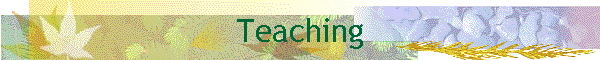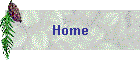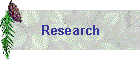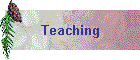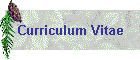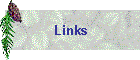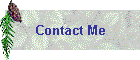My teaching experience includes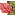Full Professor (Claremont Mckenna College) September 2018 ~

Math 32 Calculus III

Math 60C Linear Algebra with Computing

Math 111 ODE

Math 165 Numerical Analysis

Math 180 Partial Differential EquationsAssociate Professor (Claremont Mckenna College) Aug. 2011 ~ Aug. 2018

Math 461 Level Set Methods/span>

Math 31 Calculus (II)

Math 163 Numerical Analysis

Math 31 Calculus (II)

Math 111 Ordinary Differential Equations

Math 32 Calculus (III)

Math 182 Partial Differential Equations

Math 31 Calculus (II)

Math 111 Ordinary Differential Equations(National Taiwanese University) Jun. 2011

Summer School Introduction to Shape and Topology Optimization Involving Eigenvalues and EigenfunctionsAssistant Professor / Associate Professor (The Ohio State University) Sept. 2006 ~ Jun. 2011

Math 865 Mathematical Modeling of Biological Processes

Math 809 Numerical Methods for Partial Differential Equations (III)

MBI Winter 2011 Course Numerical methods for partial differential equations and their applications in biology

Math 350 Introduction to Mathematical Biology

Math 415 Ordinary and Partial Differential Equations (III)

Math 865L Mathematical Modeling of Biological Processes

Math 809 Numerical Methods for Partial Differential Equations (III)

Math 865L Mathematical Modeling of Biological Processes

Math 807 Numerical Methods for Partial Differential Equations (I)

Math 865 Topics in Applied Mathematics: Mathematical Problems in Image Processing: Partial Differential Equations and the Calculus of Variations

Math 415 Ordinary and Partial Differential Equations

Math 809 Numerical Methods for Partial Differential Equations (III)

Math 572 Linear Algebra for Applications (II)

Math 571 Linear Algebra for Applications (I)Teaching Assistant /Associate (UCLA) Apr. 2000 ~ Mar. 2002

Math 31B Calculus and Analytic Geometry

Math 32A & 32B Calculus of Several Variables

Math 61 Introduction to Discrete Structures

Math 135A & 135B Ordinary Differential Equations

Math 151B Applied Numerical Methods

Math 266A Applied Ordinary Differential EquationsTeaching Assistant (National Taiwan University) Sept. 1997 ~ Jun. 1999Teacher of Li-Wei Liu Math Center (Taiwan) Nov. 1993 ~ Jun. 1998